#### Nature acts twice

A convenient, alternative formulation can be given by allowing nature to act twice:

1. First, a nature action,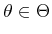, is chosen but is unknown to the robot.
2. Following this, a nature observation action is chosen to interfere with the robot's ability to sense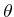.
Let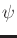denote a nature observation action, which is chosen from a nature observation action space,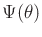. A sensor mapping,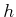, can now be defined that yields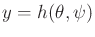for eachand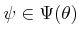. Thus, for each of the two kinds of nature actions,and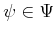, an observation,, is given. This yields an alternative way to express Formulation 9.5:

Formulation 9..6 (Nature Interferes with the Observation)
1. A nonempty, finite set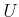called the action space.
2. A nonempty, finite set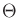called the nature action space.
3. A nonempty, finite set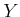called the observation space.
4. For each, a nonempty setcalled the nature observation action space.
5. A sensor mapping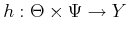.
6. A function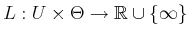called the cost function.

This nicely unifies the nondeterministic and probabilistic models with a single function. To express a nondeterministic model, it is assumed that anyis possible. Using,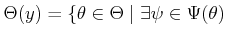such that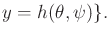(9.27)

For a probabilistic model, a distribution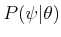is specified (often, this may reduce to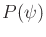). Suppose that when the domain ofis restricted to some, then it forms an injective mapping from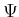to. In other words, every nature observation action leads to a unique observation, assumingis fixed. Usingand,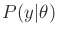is derived as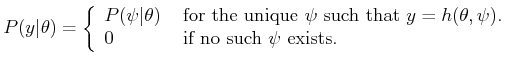(9.28)

If the injective assumption is lifted, thenis replaced by a sum over allfor which. In Formulation 9.6, the only difference between the nondeterministic and probabilistic models is the characterization of, which represents a kind of measurement interference. A strategy still takes the form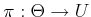. A hybrid model is even possible in which one nature action is modeled nondeterministically and the other probabilistically.

Steven M LaValle 2020-08-14